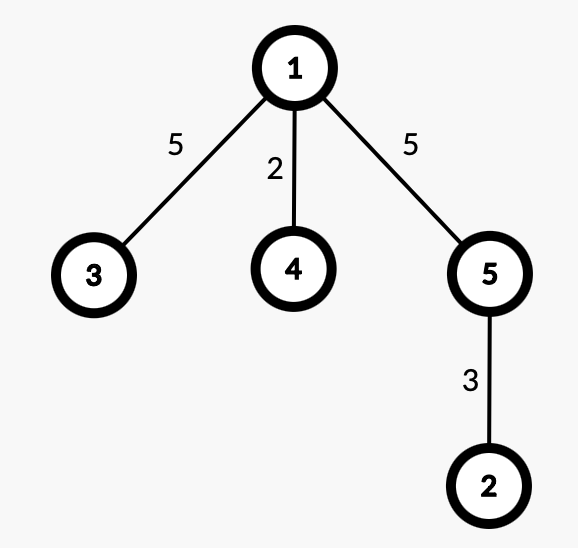# 2022 年上海市大学生程序设计竞赛 - 十二月赛

F. XY_cpp's Tree

XY_cpp has a tree with $n$ vertices, and each edge has a value. $\textcolor{black}{\rm{t}}\textcolor{red}{\rm{arjen}}$ wants to push XY_cpp $q$ times. Each time he will give XY_cpp a number $m$, and then selects $k$ vertices from the tree. He wants XY_cpp to tell him how many pairs of vertices are Good in the given vertices.

For pair $(u,v)$, we call it Good if the $\gcd$(great common divisor) of all the values on the simple path from $u$ to $v$ is less than or equal to $m$.

Howerver, XY_cpp is too vegetable. All he can do is keeping silent under $\textcolor{black}{\rm{t}}\textcolor{red}{\rm{arjen}}$‘s pushing.

### 输入格式

The first line contains one integer $n\ (1\le n\le10^5)$, the number of vertices in the tree.

Each of the next $n-1$ lines contains three integers $u,v,w\ (1\le u,v\le n,u\neq v,1\le w\le10^5)$, denoting that there is an edge with value $w$ between vertices $u$ and $v$

the next line contains one integer $q\ (1\le q\le 10^5)$, denoting the number of the queries.

Each query has two lines.

The first line contains two integers $m\ (1\le m \le 10^5)$ and $k\ (\sum k\le 10^5)$, denoting the given number and the number of selected vertices.

The second line contains $k$ distinct integers, denoting the chosen vertices.

### 输出格式

$q$ lines, each line has one integer.

The $i$th line denots the answer to the $i$th query.

### 样例

Input
5
4 1 2
5 1 5
3 1 5
2 5 3
2
3 3
5 2 1
3 2
5 3

Output
2
0

Input
10
10 1 3
7 1 4
9 7 3
5 1 4
2 7 10
4 9 9
8 2 9
3 8 6
6 8 7
2
5 3
6 7 3
9 7
6 9 10 7 4 3 2

Output
3
20


### 提示

The tree in sample $1$ is shown in picture.For query $1$: $m=3$, $k=3$. Vertices $1,2,5$ are selected. For $\rm{pair}(1,2)$, the value on their simple road are $3,5$, so their $\gcd$ is $1$. Similarly, the answer to $\rm{pair}(1,5)$ is $5$, the answer to $\rm{pair}(2,5)$ is $3$. So $\rm{pair}(1,2)$, $\rm{pair}(2,5)$ are called Good.

For query $2$: $m=3$, $k=2$. Vertices $3,5$ are selected. The values on their simple path are all $5$, so the answer to $\rm{pair}(3,5)$ is $5$, which is greater than $m$. Hence, no vertice is called good.

NaN